## Graphical display

The elements of the graphical display are: the reference point and the graphical context, the colors, the drawings, the filling pattern of closed forms, the texts and the bitmaps.

### Reference point and graphical context

The Graphics library manages a unique main window. The coordinates of the reference point of the window range from point (0,0) at the bottom left to the upper right corner of the window. The main functions on this window are:

• open_graph, of type string -> unit, which opens a window;
• close_graph, of type unit -> unit, which closes it;
• clear_graph, of type unit -> unit, which clears it.
The dimensions of the graphical window are given by the functions size_x and size_y.

The string argument of the function open_graph depends on the window system of the machine on which the program is executed and is therefore not platform independent. The empty string, however, opens a window with default settings. It is possible to specify the size of the window: under X-Windows, `" 200x300"` yields a window which is 200 pixels wide and 300 pixels high. Beware, the space at the beginning of the string `" 200x300"` is required!

The graphical context contains a certain number of readable and/or modifiable parameters:
 the current point: current_point : unit -> int * int moveto : int -> int -> unit the current color: set_color : color -> unit the width of lines: set_line_width : int -> unit the current character font: set_font : string -> unit the size of characters: set_text_size : int -> unit

### Colors

Colors are represented by three bytes: each stands for the intensity value of a main color in the RGB-model (red, green, blue), ranging from a minimum of 0 to a maximum of 255. The function rgb (of type int -> int -> int -> color) allows the generation of a new color from these three components. If the three components are identical, the resulting color is a gray which is more or less intense depending on the intensity value. Black corresponds to the minimum intensity of each component (`0`` ``0`` ``0`) and white is the maximum (`2``5``5`` ``2``5``5`` ``2``5``5`). Certain colors are predefined: black, white, red, green, blue, yellow, cyan and magenta.

The variables foreground and background correspond to the color of the fore- and the background respectively. Clearing the screen is equivalent to filling the screen with the background color.

A color (a value of type color) is in fact an integer which can be manipulated to, for example, decompose the color into its three components (from_rgb) or to apply a function to it that inverts it (inv_color).
````(* color == R * 256 * 256  +  G * 256  +  B  *)`# let` `from_rgb` `(c` ``:`` `Graphics.color)` `` ``=`` `` `` `` `let` `r` ``=`` `c` ``/`` ``6``5``5``3``6`` `` `and` `` `g` ``=`` `c` ``/`` ``2``5``6`` `mod` ``2``5``6`` `` `and` `` `b` ``=`` `c` `mod` ``2``5``6`` `` `` `` `in` `(r`,`g`,`b);;`val from_rgb : Graphics.color -> int * int * int = <fun>`# let` `inv_color` `(c` ``:`` `Graphics.color)` ``=`` `` `` `` `` `let` `(r`,`g`,`b)` ``=`` `from_rgb` `c` `` `` `` `` `in` `Graphics.rgb` `(`2``5``5``-`r)` `(`2``5``5``-`g)` `(`2``5``5``-`b);;`val inv_color : Graphics.color -> Graphics.color = <fun>`

```

The function point_color, of type int -> int -> color, returns the color of a point when given its coordinates.

### Drawing and filling

A drawing function draws a line on the screen. The line is of the current width and color. A filling function fills a closed form with the current color. The various line- and filling functions are presented in figure 5.1.

 drawing filling type plot int -> int -> unit lineto int -> int -> unit fill_rect int -> int -> int -> int -> unit fill_poly ( int * int) array -> unit draw_arc fill_arc int -> int -> int -> int -> int -> unit draw_ellipse fill_ellipse int -> int -> int -> int -> unit draw_circle fill_circle int -> int -> int -> unit

Figure 5.1: Drawing- and filling functions.

Beware, the function lineto changes the position of the current point to make drawing of vertices more convenient.

##### Drawing polygons
To give an example, we add drawing primitives which are not predefined. A polygon is described by a table of its vertices.
```# let` `draw_rect` `x0` `y0` `w` `h` ``=`` `` `` `` `let` `(a`,`b)` ``=`` `Graphics.current_point()` `` `` `` `and` `x1` ``=`` `x0`+`w` `and` `y1` ``=`` `y0`+`h` `` `` `` `in` `` `` `` `` `Graphics.moveto` `x0` `y0;` `` `` `` `` `` `Graphics.lineto` `x0` `y1;` `Graphics.lineto` `x1` `y1;` `` `` `` `` `` `` `Graphics.lineto` `x1` `y0;` `Graphics.lineto` `x0` `y0;` `` `` `` `` `` `Graphics.moveto` `a` `b;;`val draw_rect : int -> int -> int -> int -> unit = <fun>`# let` `draw_poly` `r` ``=`` `` `` `let` `(a`,`b)` ``=`` `Graphics.current_point` `()` `in` `` `` `` `let` `(x0`,`y0)` ``=`` `r`.`(`0`)` `in` `Graphics.moveto` `x0` `y0;` `` `` `` `` `` `for` `i` ``=`` ``1`` `to` `(Array.length` `r)`-``1`` `do` `` `` `` `` `` `` `let` `(x`,`y)` ``=`` `r`.`(i)` `in` `Graphics.lineto` `x` `y` `` `` `` `` `done;` `` `` `` `` `Graphics.lineto` `x0` `y0;` `` `` `` `` `Graphics.moveto` `a` `b;;`val draw_poly : (int * int) array -> unit = <fun>`

```

Please note that these functions take the same arguments as the predefined ones for filling forms. Like the other functions for drawing forms, they do not change the current point.

##### Illustrations in the painter's model
This example generates an illustration of a token ring network (figure 5.2). Each machine is represented by a small circle. We place the set of machines on a big circle and draw a line between the connected machines. The current position of the token in the network is indicated by a small black disk.

The function net_points generates the coordinates of the machines in the network. The resulting data is stored in a table.
```# let` `pi` ``=`` ``3``.``1``4``1``5``9``2``7`;;`val pi : float = 3.1415927`# let` `net_points` `(x`,`y)` `l` `n` ``=`` `` `` `` `let` `a` ``=`` ``2``.`` ``*.`` `pi` ``/.`` `(float` `n)` `in` `` `` `let` `rec` `aux` `(xa`,`ya)` `i` ``=`` `` `` `` `` `` `if` `i` ``>`` `n` `then` `[]` `` `` `` `` `` `else` `` `` `` `` `` `` `` `let` `na` ``=`` `(float` `i)` ``*.`` `a` `in` `` `` `` `` `` `` `` `let` `x1` ``=`` `xa` ``+`` `(int_of_float` `(` `cos(na)` ``*.`` `l))` `` `` `` `` `` `` `and` `y1` ``=`` `ya` ``+`` `(int_of_float` `(` `sin(na)` ``*.`` `l))` `` `in` `` `` `` `` `` `` `let` `np` ``=`` `(x1`,`y1)` `in` `` `` `` `` `` `` `` `` `np::(aux` `np` `(i`+``1`))` `` `` `` `in` `Array.of_list` `(aux` `(x`,`y)` ``1`);;`val net_points : int * int -> float -> int -> (int * int) array = <fun>`

```

The function draw_net displays the connections, the machines and the token.
```# let` `draw_net` `(x`,`y)` `l` `n` `sc` `st` ``=`` `` `` `let` `r` ``=`` `net_points` `(x`,`y)` `l` `n` `in` `` `` `` `` `draw_poly` `r;` `` `` `` `let` `draw_machine` `(x`,`y)` ``=`` `` `` `` `` `` `` `Graphics.set_color` `Graphics.background;` `` `` `` `` `` `` `Graphics.fill_circle` `x` `y` `sc;` `` `` `` `` `` `` `Graphics.set_color` `Graphics.foreground;` `` `` `` `` `` `` `Graphics.draw_circle` `x` `y` `sc` `` `` `` `in` `` `` `` `` `` `` `Array.iter` `draw_machine` `r;` `` `` `` `` `` `Graphics.fill_circle` `x` `y` `st;;`val draw_net : int * int -> float -> int -> int -> int -> unit = <fun>`

```
```

```

The following function call corresponds to the left drawing in figure 5.2.
```# draw_net` `(`1``4``0``,``2``0`)` ``6``0``.``0`` ``1``0`` ``1``0`` ``3`;;`- : unit = ()`

```
```# save_screen` ``"IMAGES/tokenring.caa"`;;`- : unit = ()`

```
```

```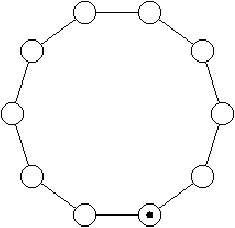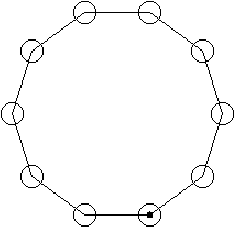Figure 5.2: Tokenring network.

We note that the order of drawing objects is important. We first plot the connections then the nodes. The drawing of network nodes erases some part of the connecting lines. Therefore, there is no need to calculate the point of intersection between the connection segments and the circles of the vertices. The right illustration of figure 5.2 inverts the order in which the objects are displayed. We see that the segments appear inside of the circles representing the nodes.

### Text

The functions for displaying texts are rather simple. The two functions draw_char (of type char -> unit) and draw_string (of type string -> unit) display a character and a character string respectively at the current point. After displaying, the latter is modified. These functions do not change the current font and its current size.

### Note

The displaying of strings may differ depending on the graphical interface.

The function text_size takes a string as input and returns a pair of integers that correspond to the dimensions of this string when it is displayed in the current font and size.

##### Displaying strings vertically
This example describes the function draw_string_v, which displays a character string vertically at the current point. It is used in figure 5.3. Each letter is displayed separately by changing the vertical coordinate.
```# let` `draw_string_v` `` `s` ``=`` `` `` `` `let` `(xi`,`yi)` ``=`` `Graphics.current_point()` `` `` `` `and` `l` ``=`` `String.length` `s` `` `` `` `and` `(`_,`h)` ``=`` `Graphics.text_size` `s` `` `` `` `in` `` `` `` `` `` `` `Graphics.draw_char` `s`.[``0``]`;` `` `` `` `` `` `for` `i`=``1`` `to` `l`-``1`` `do` `` `` `` `` `` `` `` `let` `(`_,`b)` ``=`` `Graphics.current_point()` `` `` `` `` `` `` `` `` `in` `Graphics.moveto` `xi` `(b`-`h);` `` `` `` `` `` `` `` `` `` `` `Graphics.draw_char` `s`.[`i`]`` `` `` `` `` `` `` `` `done;` `` `` `` `` `let` `(a`,_`)` ``=`` `Graphics.current_point()` `in` `Graphics.moveto` `a` `yi;;`val draw_string_v : string -> unit = <fun>`

```
This function modifies the current point. After displaying, the point is placed at the initial position offset by the width of one character.

The following program permits displaying a legend around the axes (figure 5.3)
```#` `Graphics.moveto` ``0`` ``1``5``0`;` `Graphics.lineto` ``3``0``0`` ``1``5``0`;` `Graphics.moveto` ``2`` ``1``3``0`;` `Graphics.draw_string` ``"abscissa"`;` `Graphics.moveto` ``1``5``0`` ``0`;` `Graphics.lineto` ``1``5``0`` ``3``0``0`;` `Graphics.moveto` ``1``3``5`` ``2``8``0`;` `draw_string_v` ``"ordinate"`;;`- : unit = ()`

```Figure 5.3: Legend around axes.

If we wish to realize vertical displaying of text, it is necessary to account for the fact that the current point is modified by the function draw_string_v. To do this, we define the function draw_text_v, which accepts the spacing between columns and a list of words as parameters.
```# let` `draw_text_v` `n` `l` ``=`` `` `` `` `let` `f` `s` ``=`` `let` `(a`,`b)` ``=`` `Graphics.current_point()` `` `` `` `` `` `` `` `` `` `` `` `` `` `in` `draw_string_v` `s;` `` `` `` `` `` `` `` `` `` `` `` `` `` `` `` `Graphics.moveto` `(a`+`n)` `b` `` `` `in` `List.iter` `f` `l;;`val draw_text_v : int -> string list -> unit = <fun>`

```

If we need further text transformations like, for example, rotation, we will have to take the bitmap of each letter and perform the rotation on this set of pixels.

### Bitmaps

A bitmap may be represented by either a color matrix (color array array) or a value of abstract type 1 image, which is declared in library Graphics. The names and types of the functions for manipulating bitmaps are given in figure 5.4.

 function type make_image color array array -> image dump_image image -> color array array draw_image image -> int -> int -> unit get_image int -> int -> int -> int -> image blit_image image -> int -> int -> unit create_image int -> int -> image

Figure 5.4: Functions for manipulating bitmaps.

The functions make_image and dump_image are conversion functions between types image and color array array. The function draw_image displays a bitmap starting at the coordinates of its bottom left corner.

The other way round, one can capture a rectangular part of the screen to create an image using the function get_image and by indicating the bottom left corner and the upper right one of the area to be captured. The function blit_image modifies its first parameter (of type image) and captures the region of the screen where the lower left corner is given by the point passed as parameter. The size of the captured region is the one of the image argument. The function create_image allows initializing images by specifying their size to use them with blit_image.

The predefined color transp can be used to create transparent points in an image. This makes it possible to display an image within a rectangular area only; the transparent points do not modify the initial screen.

##### Polarization of Jussieu
This example inverts the color of points of a bitmap. To do this, we use the function for color inversion presented on page ??, applying it to each pixel of a bitmap.
```# let` `inv_image` `i` ``=`` `` `` `` `let` `inv_vec` ``=`` `Array.map` `(fun` `c` `->` `inv_color` `c)` `in` `` `` `let` `inv_mat` ``=`` `Array.map` `inv_vec` `in` `` `` `` `let` `inverted_matrix` ``=`` `inv_mat` `(Graphics.dump_image` `i)` `in` `` `` `` `Graphics.make_image` `inverted_matrix;;`val inv_image : Graphics.image -> Graphics.image = <fun>`

```

Given the bitmap jussieu, which is displayed in the left half of figure 5.5, we use the function inv_image and obtain a new ``solarized'' bitmap, which is displayed in the right half of the same figure.

```# let` `f_jussieu2` `()` ``=`` `inv_image` `jussieu1;;` ``val f_jussieu2 : unit -> Graphics.image = <fun>`

```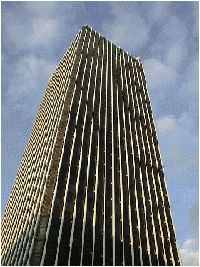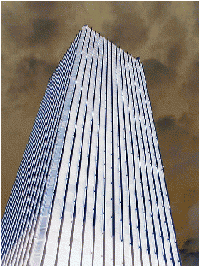Figure 5.5: Inversion of Jussieu.

### Example: drawing of boxes with relief patterns

In this example we will define a few utility functions for drawing boxes that carry relief patterns. A box is a generic object that is useful in many cases. It is inscribed in a rectangle which is characterized by a point of origin, a height and a width.

 To give an impression of a box with a relief pattern, it is sufficient to surround it with two trapezoids in a light color and two others in a somewhat darker shade.Inverting the colors, one can give the impression that the boxes are on top or at the bottom.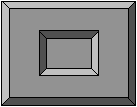##### Implementation
We add the border width, the display mode (top, bottom, flat) and the colors of its edges and of its bottom. This information is collected in a record.
```# type` `relief` ``=`` `Top` ``|`` `Bot` ``|`` `Flat;;# type` `box_config` ``=`` `` `{` `x`:`int;` `y`:`int;` `w`:`int;` `h`:`int;` `bw`:`int;` `mutable` `r`:`relief;` `` `` `` `b1_col` ``:`` `Graphics.color;` `` `` `` `b2_col` ``:`` `Graphics.color;` `` `` `` `b_col` ``:`` `Graphics.color};;

```
Only field r can be modified. We use the function draw_rect defined at page ??, which draws a rectangle.

For convenience, we define a function for drawing the outline of a box.
```# let` `draw_box_outline` `bcf` `col` ``=`` `` `` `Graphics.set_color` `col;` `` `draw_rect` `bcf`.`x` `bcf`.`y` `bcf`.`w` `` `bcf`.`h;;`val draw_box_outline : box_config -> Graphics.color -> unit = <fun>`

```

The function of displaying a box consists of three parts: drawing the first edge, drawing the second edge and drawing the interior of the box.
```# let` `draw_box` `bcf` ``=`` `` `` `` `let` `x1` ``=`` `bcf`.`x` `and` `y1` ``=`` `bcf`.`y` `in` `` `` `let` `x2` ``=`` `x1`+`bcf`.`w` `and` `y2` ``=`` `y1`+`bcf`.`h` `in` `` `` `` `let` `ix1` ``=`` `x1`+`bcf`.`bw` `and` `ix2` ``=`` `x2`-`bcf`.`bw` `` `` `` `and` `iy1` ``=`` `y1`+`bcf`.`bw` `and` `iy2` ``=`` `y2`-`bcf`.`bw` `in` `` `` `` `let` `border1` `g` ``=`` `` `` `` `` `Graphics.set_color` `g;` `` `` `` `` `Graphics.fill_poly` `` `` `` `` `` `` `` ``[|`` `(x1`,`y1);(ix1`,`iy1);(ix2`,`iy1);(ix2`,`iy2);(x2`,`y2);(x2`,`y1)` ``|]`` `` `` `` `in` `` `` `let` `border2` `g` ``=`` `` `` `` `` `` `Graphics.set_color` `g;` `` `` `` `` `Graphics.fill_poly` `` `` `` `` `` `` `` ``[|`` `(x1`,`y1);(ix1`,`iy1);(ix1`,`iy2);(ix2`,`iy2);(x2`,`y2);(x1`,`y2)` ``|]`` `` `` `in` `` `` `Graphics.set_color` `bcf`.`b_col;` `` `` `(` `match` `bcf`.`r` `with` `` `` `` `` `` `` `` `` `Top` `` `->` `` `` `` `` `` `` `` `` `` `` `` `Graphics.fill_rect` `ix1` `iy1` `(ix2`-`ix1)` `(iy2`-`iy1);` `` `` `` `` `` `` `` `` `` `` `border1` `bcf`.`b1_col;` `` `` `` `` `` `` `` `` `` `` `` `border2` `bcf`.`b2_col` `` `` `` `` `` `` ``|`` `Bot` `` `->` `` `` `` `` `` `` `` `` `` `` `` `Graphics.fill_rect` `ix1` `iy1` `(ix2`-`ix1)` `(iy2`-`iy1);` `` `` `` `` `` `` `` `` `` `` `border1` `bcf`.`b2_col;` `` `` `` `` `` `` `` `` `` `` `` `border2` `bcf`.`b1_col` `` `` `` `` `` `` ``|`` `Flat` `->` `` `` `` `` `` `` `` `` `` `` `` `Graphics.fill_rect` `x1` `y1` `bcf`.`w` `bcf`.`h` `);` `` `` `draw_box_outline` `bcf` `Graphics.black;;`val draw_box : box_config -> unit = <fun>`

```

The outline of boxes is highlighted in black. Erasing a box fills the area it covers with the background color.
```# let` `erase_box` `bcf` ``=`` `` `` `` `` `Graphics.set_color` `bcf`.`b_col;` `` `` `` `` `Graphics.fill_rect` `(bcf`.`x`+`bcf`.`bw)` `(bcf`.`y`+`bcf`.`bw)` `` `` `` `` `` `` `` `` `` `` `` `` `` `` `` `` `` `` `` `` `` `` `` `(bcf`.`w`-`(`2``*`bcf`.`bw))` `(bcf`.`h`-`(`2``*`bcf`.`bw));;`val erase_box : box_config -> unit = <fun>`

```

Finally, we define a function for displaying a character string at the left, right or in the middle of the box. We use the type position to describe the placement of the string.
```# type` `position` ``=`` `Left` ``|`` `Center` ``|`` `Right;;` ``type position = | Left | Center | Right`# let` `draw_string_in_box` `pos` `str` `bcf` `col` ``=`` `` `` `` `` `` `let` `(w`,`` `h)` ``=`` `Graphics.text_size` `str` `in` `` `` `` `` `let` `ty` ``=`` `bcf`.`y` ``+`` `(bcf`.`h`-`h)`/``2`` `in` `` `` `` `` `` `(` `match` `pos` `with` `` `` `` `` `` `` `` `` `` `` `` `Center` `->` `Graphics.moveto` `(bcf`.`x` ``+`` `(bcf`.`w`-`w)`/``2`)` `ty` `` `` `` `` `` `` `` `` `` ``|`` `Right` `` `->` `let` `tx` ``=`` `bcf`.`x` ``+`` `bcf`.`w` ``-`` `w` ``-`` `bcf`.`bw` ``-`` ``1`` `in` `` `` `` `` `` `` `` `` `` `` `` `` `` `` `` `` `` `` `` `` `` `Graphics.moveto` `tx` `ty` `` `` `` `` `` `` `` `` `` ``|`` `Left` `` `` `->` `let` `tx` ``=`` `bcf`.`x` ``+`` `bcf`.`bw` ``+`` ``1`` `in` `Graphics.moveto` `tx` `ty` `` `);` `` `` `` `` `Graphics.set_color` `col;` `` `` `` `` `Graphics.draw_string` `str;;`val draw_string_in_box :``  position -> string -> box_config -> Graphics.color -> unit = <fun>`

```

##### Example: drawing of a game
We illustrate the use of boxes by displaying the position of a game of type ``tic-tac-toe'' as shown in figure 5.6. To simplify the creation of boxes, we predefine colors.
```# let` `set_gray` `x` ``=`` `` `(Graphics.rgb` `x` `x` `x);;`val set_gray : int -> Graphics.color = <fun>`# let` `gray1`=`` `set_gray` ``1``0``0`` `and` `gray2`=`` `set_gray` ``1``7``0`` `and` `gray3`=`` `set_gray` ``2``4``0`;;`val gray1 : Graphics.color = 6579300``val gray2 : Graphics.color = 11184810``val gray3 : Graphics.color = 15790320`

```

We define a function for creating a grid of boxes of same size.
```# let` `rec` `create_grid` `nb_col` `n` `sep` `b` `` ``=`` `` `` `if` `n` ``<`` ``0`` `then` `[]` `` `` `else` `` `` `` `` `` `let` `px` ``=`` `n` `mod` `nb_col` `and` `py` ``=`` `n` ``/`` `nb_col` `in` `` `` `` `` `let` `nx` ``=`` `b`.`x` ``+`sep` ``+`` `px`*`(b`.`w`+`sep)` `` `` `` `` `and` `ny` ``=`` `b`.`y` ``+`sep` ``+`` `py`*`(b`.`h`+`sep)` `in` `` `` `` `` `let` `b1` ``=`` `{b` `with` `x`=`nx;` `y`=`ny}` `in` `` `` `` `` `b1::(create_grid` `nb_col` `(n`-``1`)` `sep` `b);;`val create_grid : int -> int -> int -> box_config -> box_config list = <fun>`

```

And we create the vector of boxes:
```# let` `vb` ``=`` `` `` `` `let` `b` ``=`` `` `{x`=``0`;` `y`=``0`;` `w`=``2``0`;h`=``2``0`;` `bw`=``2`;` `` `` `` `` `` `` `` `` `` `` `` `` `b1_col`=`gray1;` `b2_col`=`gray3;` `b_col`=`gray2;` `r`=`Top}` `in` `` `` `` `Array.of_list` `(create_grid` ``5`` ``2``4`` ``2`` `` `b);;`val vb : box_config array =``  [|{x=90; y=90; w=20; h=20; bw=2; r=Top; b1_col=6579300; b2_col=15790320;``     b_col=11184810};``    {x=68; y=90; w=20; h=20; bw=2; r=Top; b1_col=6579300; b2_col=15790320;``     b_col=...};``    ...|]`

```

Figure 5.6 corresponds to the following function calls:

```# Array.iter` `draw_box` `vb;` `draw_string_in_box` `Center` ``"X"`` `vb`.`(`5`)` `Graphics.black;` `draw_string_in_box` `Center` ``"X"`` `vb`.`(`8`)` `Graphics.black;` `draw_string_in_box` `Center` ``"O"`` `vb`.`(`1``2`)` `Graphics.yellow;` `draw_string_in_box` `Center` ``"O"`` `vb`.`(`1``1`)` `Graphics.yellow;;`- : unit = ()`

```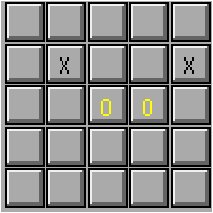Figure 5.6: Displaying of boxes with text.